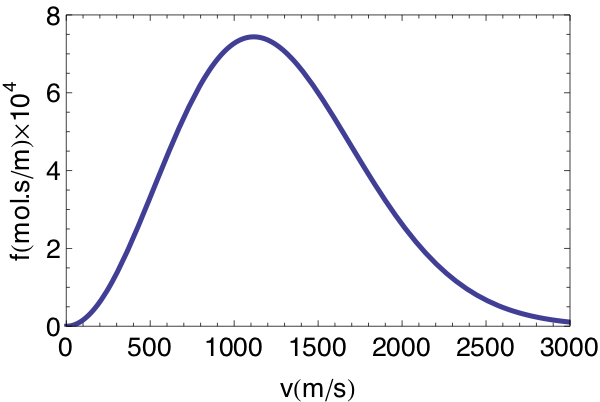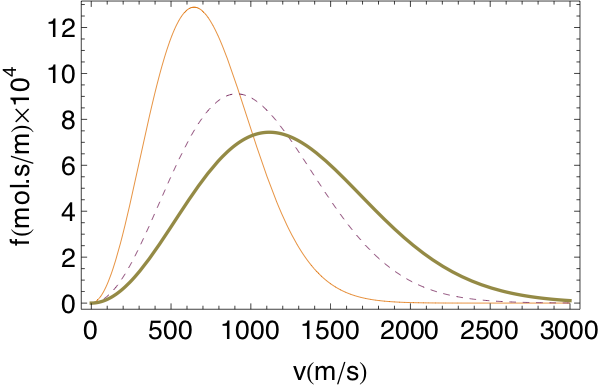## Section27.2Maxwell's Distribution of Molecular Speeds

The molecules of an ideal gas move independently of each other. When a molecule bounces off a wall, its speed does not change because we assume that the collision with the walls are elastic. Furthermore, since the molecules of an ideal gas do not interact with one another, there will also be no collision among the molecules of an ideal gas. Therefore, speeds of molecules of ideal gas remain constant in time.

But, molecules of real gases do interact and collide with each other and with the walls of the container, resulting in the change of velocity and speed upon collision. Therefore even if you start out by giving the same speeds to all the molecules, the speeds will soon become different in a real gas.

James Clerk Maxwell studied this problem and found a formula for the distribution of speeds, shown in Figure 27.2.1, but his results apply only to ideal gases, whose molecules can be allowed to collide with one another as billiard balls. Since most real gases, when they are dilute or at high temperature, behave like an ideal gas, the distribution function worked out by Maxwell can be used also for understanding real gases.Figure 27.2.1. Maxwell molecular speed distribution in one mole of helium at 300 K. The distribution function was multiplied by $10^4$ for plotting.

The mean of this distribution gives the average speed and it can be shown that this depends on the temperarure $T\text{,}$ in kelvin scale, and the molecular mass $m\text{.}$

\begin{equation} v_\text{ave} = \sqrt{\dfrac{8k_B T}{\pi m}}.\tag{27.2.1} \end{equation}

We can also write this in molar quantities by multiplying and dividing the expression on the right side by the Avogadro number $N_A\text{.}$

\begin{equation} v_\text{ave} = \sqrt{\dfrac{8R T}{\pi M}},\tag{27.2.2} \end{equation}

where $M=N_A m$ is the molecular weight and $R$ the gas constant. The peak of the distribution does not occur at the average speed. The peak occurs at, what is called, the the most probably speed, $v_p$

\begin{equation} v_p = \sqrt{ \dfrac{2k_BT}{m} },\tag{27.2.3} \end{equation}

which is actually less than the average speed since the distribution has a long tail.

Since kinetic energy varies as square of speed, another type of average speed based on taking square-root of the average of speed squared gives us more accurate information about the energetics of the gas moleculs. This average is called the root mean squared or the rms speed. The rms speed of Maxwell's distribution is

\begin{equation} v_\text{rms} = \sqrt{\dfrac{3k_BT}{m}}.\tag{27.2.4} \end{equation}

The average kinetic energy of a monatomic ideal gas, such as Helium, is

\begin{equation} \text{KE} = \dfrac{1}{2} m v_\text{rms}^2 = \dfrac{3}{2} k_B T.\tag{27.2.5} \end{equation}

The factor 3 on the right side can be understood to correspond to three different directions a molecule can move, i.e., degrees of freedom of the molecule, and the quantity $(\dfrac{1}{2} k_B T)$ is the kinetic energy for each degree of freedom.

\begin{equation} \text{KE per degree of freedom} = \dfrac{1}{2} k_B T.\tag{27.2.6} \end{equation}

For a datomic molecular gas, such as Nitrogen, the average kinetic energy would be different when expressed in temperature.

\begin{equation} \text{KE} = \dfrac{1}{2} m v_\text{rms}^2 = \dfrac{5}{2} k_B T.\tag{27.2.7} \end{equation}

The difference in the factor between the monatomic and diatomic comes from the difference in degrees of freedom of each molecule. Monatomic molecule has three translational degrees of freedom and diatomic molecule has three translational, one vibrational, and one rotational degree of freedom, making it altogether five degrees of freedom. For triatomic molecules, such as water molecule, there are additional degrees of freedoms.

Find (a) the average speed and (b) the root-mean squared speed of molecules of nitrogen gas treated as an ideal gas at room temperature $27^{\circ}\text{C}\text{.}$

Data: Avogadro's number, $N_A = 6.022\times 10^{23}\text{,}$ Boltzmann's constant, $k_B = 1.38\times 10^{-23} \text{m}^2\text{kg}\text{s}^{-2}\text{K}^{-1}\text{.}$

Hint

(a) Use formula for the average speed. Also, make sure you change temperture to kelvin. (b) Use the formula for diatomic gas.

(a) $494\text{ m/s} \text{,}$ (b) $692\text{ m/s}\text{.}$

Solution 1 (a)

(a) The average speed in molar units is

\begin{equation*} v_\text{ave} = \sqrt{\dfrac{8 k_B T}{\pi m}}. \end{equation*}

Here the numbers are

\begin{align*} \amp T = 273+27=300K.\\ \amp m = \dfrac{ 28\times 10^{-3}\text{ kg}}{6.022\times 10^{23} } = 4.32 \times 10^{-26}\text{ kg}. \end{align*}

Using these numbers we get

\begin{equation*} v_\text{ave} = 494\text{ m/s}. \end{equation*}
Solution 2 (b)

(b) For a diatomic molecular gas, the RMS speed from kinetic energy formula is given by

\begin{equation*} v_\text{rms} = \sqrt{\dfrac{5k_B T}{m}} = 692\text{ m/s}. \end{equation*}

### Subsection27.2.1(Calculus) Maxwell's distribution

Suppose there are $N$ molecules of a gas at temperature $T\text{.}$ Let the mass of each molecule be $m\text{.}$ According to Maxwell, molecules in a gas move at many different speeds, and, since the number of molecules $N$ is very large in any finite sample, the speeds of molecules can be considered to be distributed continuously, i/e., we can treat speed as a continuous real variable for mathematical purposes.

Maxwell's distribution of speeds for molecules of a gas gives the number $f(v)dv$ of molecules that have speed between $v$ and $v+dv\text{.}$

\begin{equation} f(v) dv = \alpha v^2 \exp{\left(-\frac{\frac{1}{2}mv^2}{k_BT} \right)}dv, \label{eq-max-boltz-1a}\tag{27.2.8} \end{equation}

where $\alpha$ is a normalization constant and $k_B$ is the Boltzmann constant. The normalization constant $\alpha$ is determined by requiring that the integration over all possible speeds be equal to the total number $N$ of molecules in the system.

\begin{equation} N = \int_0^\infty f(v) dv\label{}\label{eq-max-boltz-2}\tag{27.2.9} \end{equation}

By using $f(v)dv$ from Eq. (27.2.10) and integrating, we find the normalization constant $\alpha$ to be

\begin{equation*} \alpha = 4\pi N \left( \frac{m}{2\pi k_B T} \right)^{3/2}. \end{equation*}

Thus Maxwell distribution of speeds for a gas with $N$ molecules at temperature $T$

\begin{equation} f(v) dv = 4\pi N \left( \frac{m}{2\pi k_B T} \right)^{3/2} v^2 \exp{\left(-\frac{\frac{1}{2}mv^2}{k_BT} \right)}dv.\label{eq-max-boltz-1}\tag{27.2.10} \end{equation}

Note that the distribution function $f(v)$ is not equal to the number of molecules having speed $v\text{.}$ Rather, $f(v)$ is “a number density in speed space,” so that the number of molecules with speed between $v$ and $v+dv$ is given by $f(v)dv\text{.}$ The units of $f(v)$ is seconds/meter since its product with speed element $dv$ is a unitless number.

The Maxwell distribution function depends on the speed as a product of the square of the speed and an exponential function with a negative exponent containing the ratio of kinetic energy of one molecule and an energy scale $k_BT\text{.}$ Thus, the distribution of speeds critically depends upon the relative magnitudes of the kinetic energy of molecules and the energy scale $k_BT$ set by the temperature $T\text{.}$

The negative exponential factor makes it less likely that a molecule will have much higher kinetic energy than $k_BT$ while the quadratic prefactor of velocity squared makes low speeds less likely. The competition between the two factors results in a maximum for the distribution.

Figure 27.2.1 shows the distribution function $f(v)$ for the Helium gas at 300 K. Note that the distribution is not symmetric about the peak, and the most probable speed is not the same as the average speed of molecules. The lowest speed is zero, but the distribution trails off to infinity for the highest speed.

Figure 27.2.3 shows distribution of molecular speeds in the same system but at different temperatures. The peak speed of the distribution shifts to higher values for higher temperatures. Since the number of molecules is the same in the three graphs, the areas under the curves for different temperatures must be equal to each other. Therefore, as the peak speed shifts to the right the distribution curve flattens out.Figure 27.2.3. Molecular speed distributions of one mole of helium at T=100 K (thin line), T=200 K (dashed line), and T=300 K (thick line). The three distribution functions were multiplied by $10^4$ for plotting purposes. With increasing temperature, the peak speed shifts to higher speeds and the distribution becomes wider.

Find the mean of the Maxwell's distribution.

Hint

Compute $\dfrac{1}{N}\int_0^\infty\, v \, f(v)\, dv. \text{.}$

$\sqrt{\dfrac{8k_B T}{\pi m}} \text{.}$

Solution

The mean of the distribution is given by the following integral.

\begin{equation*} \langle v \rangle = \dfrac{1}{N}\int_0^\infty\, v \, f(v)\, dv. \end{equation*}

Performing this integral we get

\begin{equation*} \langle v \rangle = \sqrt{\dfrac{8k_B T}{\pi m}}. \end{equation*}

The molecules for Maxwell distribution have only translational kinetic energy. Therefore, we can express their speed $v$ in terms of energy $E$ of a molecule.

\begin{equation*} \frac{1}{2} m v^2 = E\ \ \ \text{(since PE = 0.)} \end{equation*}

(a) Change the variable from $v$ to $E$ in the Maxwell distribution and obtain the distribution function for energy from the distribution function of speed. Note that distribution function for energy, lets say $g(E)$ is a function of energy $E$ such that $g(E) dE$ gives the number of particles with energy between $E$ and $E+dE\text{.}$ The distribution of energy is called the Maxwell-Boltzmann energy distribution. Ans:

\begin{equation*} g(E) dE = \frac{2\pi N}{(\pi k_B T)^{3/2}}\sqrt{E} \exp{(-E/k_BT)} dE. \end{equation*}

(b) Show that integration of $g(E)$ from $E= 0$ to $\infty$ yields $N\text{,}$ the total number of molecules.

(c) Calculate the average energy and show it is equal to $\frac{3}{2} k_BT\text{.}$

Hint

Use $f(v) \dfrac{dv}{dE} dE.$

Solution 1 (a)

From $E=\dfrac{1}{2} m v^2 \text{,}$ we get

\begin{equation*} dE = m v dv\ \ \Longrightarrow\ \ \dfrac{dv}{dE} = \dfrac{1}{mv}. \end{equation*}

Let

\begin{equation*} \beta = \dfrac{1}{k_B T},\ \ a = \dfrac{m}{2}\,\beta,\ \ \alpha = 4N\, a^{3/2}/\sqrt{\pi}. \end{equation*}

Then, the distribution of speeds looks simpler

\begin{equation*} f(v)dv = \alpha\, v^2\, \exp(-a v^2)\, dv. \end{equation*}

We can see that the distribution in speed can be recast with $E$ as indeopendent variable by

\begin{equation*} f(v) \dfrac{dv}{dE} dE. \end{equation*}

Write this as

\begin{equation*} \alpha\, v^2\, \exp(-a v^2)\, \dfrac{1}{mv} dE. \end{equation*}

With $v = \sqrt{2E/m}$ we get the result after simplification.

\begin{equation*} \dfrac{2N}{\sqrt{\pi}}\, \beta^{3/2}\, \sqrt{E}\, \exp(-\beta E)\, dE. \end{equation*}

Therefore, the energy distribution is

\begin{equation*} g(E) dE = \dfrac{2N}{\sqrt{\pi}}\, \beta^{3/2}\, \sqrt{E}\, \exp(-\beta E)\, dE, \end{equation*}

where $\beta = 1/k_BT\text{.}$

Solution 2 (b)

(b) I looked up the following integral from wolfram alpha website with the command “integrate x^(1/2) exp(-a x) dx from x=0 to infinity” to get

\begin{equation*} \int_0^\infty \sqrt{x} \exp(-a\,x)\,dx = \dfrac{\sqrt{\pi}}{2a^{3/2}}. \end{equation*}

Using this I was able to prove the required result.

Solution 3 (c)

From definition of mean of a distribution, we will have

\begin{equation*} E_\text{ave} = \int_0^\infty E\,g(E)\, dE. \end{equation*}

This requires the following integral

\begin{equation*} \int_0^\infty E\sqrt{E} \exp(-\beta\,E)\,dE = \dfrac{3\,\pi^{1/2}}{4\beta^{5/2}}. \end{equation*}

Using this gives the required result.